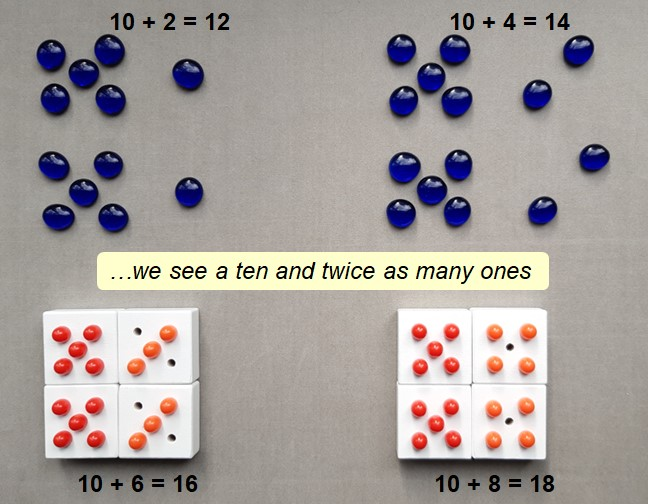## Doubles

Using dot patterns based on 5 can provide a useful visual when doubling beyond numbers greater than 5. We can see 8 represented as 5 and 3, so when we double 8, we see 10 and 6, or 16.We can also see that doubling a whole number always results in an even number.

## Near doubles

Near (or close) doubles are made when we add consecutive numbers (numbers that follow each other in the counting sequence). So 8+7 can be seen as 1 more than double 7, or 1 less than double 8.We can see that adding two consecutive numbers always results in an odd number.

Play Nearly double to practise this